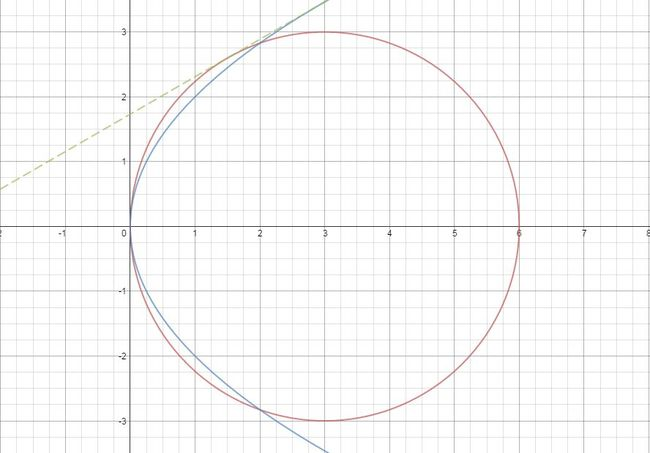# Don't find the intersectionWhich of the given equations is the common tangent to the circle ${(x-3)}^2+{y}^2=9$ and the parabola ${y}^2=4x$ touching both the conics above the $x$-axis?

×